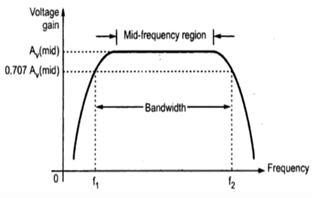Courses

# Test: General Frequency Consideration

## 11 Questions MCQ Test Analog Electronics | Test: General Frequency Consideration

Description
This mock test of Test: General Frequency Consideration for Electrical Engineering (EE) helps you for every Electrical Engineering (EE) entrance exam. This contains 11 Multiple Choice Questions for Electrical Engineering (EE) Test: General Frequency Consideration (mcq) to study with solutions a complete question bank. The solved questions answers in this Test: General Frequency Consideration quiz give you a good mix of easy questions and tough questions. Electrical Engineering (EE) students definitely take this Test: General Frequency Consideration exercise for a better result in the exam. You can find other Test: General Frequency Consideration extra questions, long questions & short questions for Electrical Engineering (EE) on EduRev as well by searching above.
QUESTION: 1

### The frequency f1 and f2 from the below picture are respectively called​Solution:

The frequencies are called lower cut-off frequency and upper cut-off frequency because at these frequencies power of the signal becomes half of its original value. So they are also called 3dB cut-off frequencies.

QUESTION: 2

### Bandwidth of amplifier is

Solution:

3dB bandwidth of an amplifier is the difference between upper cut-off frequency and lower cut-off frequency. The unity gain bandwidth is the difference between frequencies were gain is 1.

QUESTION: 3

### At 3dB cut-off frequency the voltage gain will be

Solution:

3dB cut-off frequency is the frequency at which the power becomes half of its maximum value. That is the voltage gain becomes 0.707 times maximum voltage gain.

QUESTION: 4

At 3dB cut-off frequencies power will be

Solution:

3dB cut-off frequency is the frequency at which the power becomes half of its maximum value. That is the voltage gain becomes 0.707 times maximum voltage gain. The importance of 3dB points is that for a human ear, it will not notice the fall in power of the signal up to 50% of maximum power.

QUESTION: 5

A voltage amplifier has a voltage gain of 100.What will be gain at 3dB cut-off frequencies

Solution:

3dB cut-off frequency is the frequency at which the power becomes half of its maximum value. That is the voltage gain becomes 0.707 times maximum voltage gain. Therefore if voltage gain is 100 then gain at 3dB frequencies will be
100 x 0.707 = 70.7.

QUESTION: 6

What is the roll-off rate of single order filter

Solution:

At lower and higher frequencies the gain is decreasing. This fall or decrease in gain is known as roll-off rate. It is commonly specified in dB/octave or dB/decade. For a single order filter the roll-off rate is 6dB/octave or 20dB/decade.

QUESTION: 7

-6dB is equivalent to __________ power gain

Solution:

The relation between dB and power gain is
dB = 10 log(power gain)
That is power gain for -6dB is
10(-6 /10) = 0.251.

QUESTION: 8

Voltage gain of 1,00,000 is equivalent to

Solution:

dB = 20 log(voltage gain)
Therefor 100000 voltage gain is equivalent to
20 log(1,00,000)dB = 100 dB.

QUESTION: 9

If the output power from an audio amplifier is measured at 100W when the signal frequency is 1kHz, and 1W when the signal frequency is 10kHz. Calculate the dB change in power.

Solution:

The initial power gain in dB = 10 log (output power)
= 10 log(100) = 20dB
The final power gain in dB = 10 log(output power)
= 10 log(1) = 0 dB
So change in power = final power – initial power
= 0-20 = -20dB.

QUESTION: 10

If the output power from an audio amplifier is measured at 100W when the signal frequency is 1kHz, and 1W when the signal frequency is 10kHz. Calculate the dB change in power.

Solution:

The initial power gain in dB = 10 log (output power)
= 10 log(100) = 20dB
The final power gain in dB = 10 log(output power)
= 10 log(1) = 0 dB
So change in power = final power – initial power
= 0-20 = -20dB.

QUESTION: 11

If an electronic system produces a 48mV output voltage when a 12mV signal is applied, calculate the decibel value of the systems output voltage gain

Solution:

Gain of the system is = output voltage/input voltage
= 48/12 = 4
Gain in dB = 20 log(voltage gain)
= 20 log(4) = 12.04 dB.